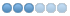# Trouble with Strchr

• Chime
•Topic Author
• Offline
• Senior Member
•3 years 4 weeks ago #7939 by Chime
Trouble with Strchr was created by Chime
Getting some weird data corruptiony-type stuff sometimes when my RPOSE command is used.

colorPose is intended to identify when a quotation mark is added to a string, then color what's between them.

The culpable function:
```char * colorPose(char *argument)
{
char *final_string;
char tempString[MAX_STRING_LENGTH];
bool inquotes = FALSE;
int offset = 0;
int x = 0;

while (x < strlen(argument))
{
if (argument[x] == '"')
{
if (inquotes == TRUE)
{
tempString[x + offset] = '@';
tempString[x + offset + 1] = 'n';
tempString[x + offset + 2] = '"';
offset += 2;
inquotes = FALSE;
}
else
{
tempString[x + offset] = '"';
tempString[x + offset + 1] = '@';
tempString[x + offset + 2] = 'W';
offset += 2;
inquotes = TRUE;
}
}
else
tempString[x + offset] = argument[x];

x++;
}
if (inquotes == TRUE)
{
tempString[x + offset] = '"';
tempString[x + offset + 1] = '@';
tempString[x + offset + 2] = 'n';
offset += 3;
inquotes = FALSE;
}

if (CONFIG_SPECIAL_IN_COMM && legal_communication(tempString))
parse_at(tempString);

tempString[x + offset] = '\0';
final_string = tempString;
return final_string;
}```

Called in do_rpose (Maybe this is the problem portion, instead of the function itself?):
```if (strchr(argument, '"') != NULL)
argument = colorPose(argument);```

Testing shows this, for example of the weird:

rpecho Chime shrieks, "Why though?"

I think it's time to acknowledge I'm nowhere near clever enough, a few hours of bashing at the function considered, to detect what the trouble is without a more educated eye.

Is there an issue I'm missing?

• thomas
•• Offline
•3 years 3 weeks ago #7941 by thomas
Replied by thomas on topic Trouble with Strchr
Move this line above the call to parse_at:
` tempString[x + offset] = '\0';`
The following user(s) said Thank You: Chime

•Topic Author
•